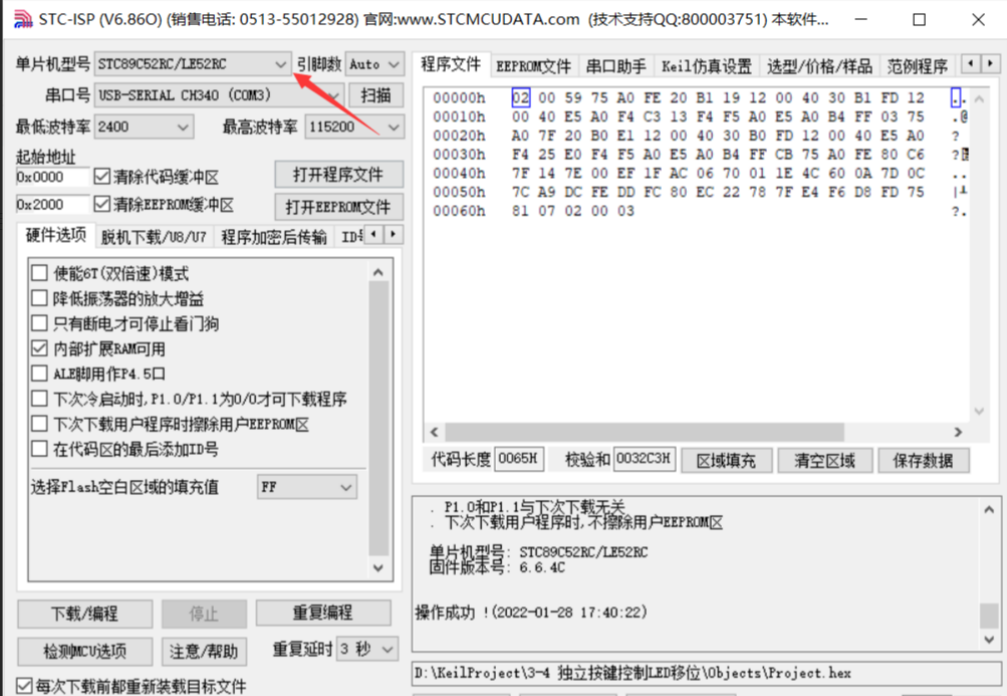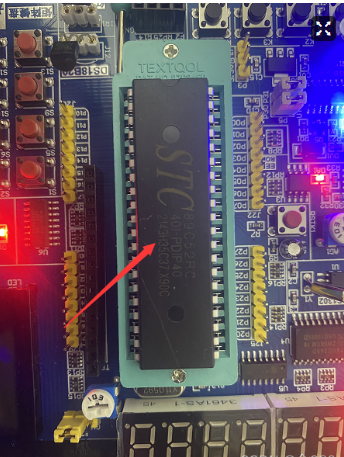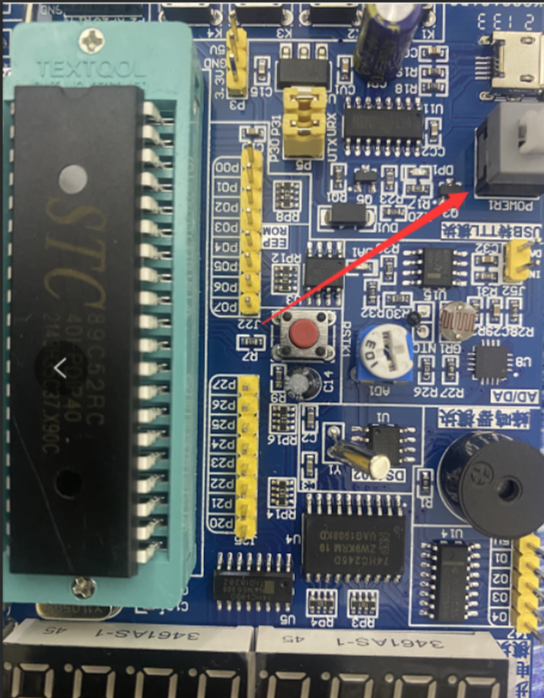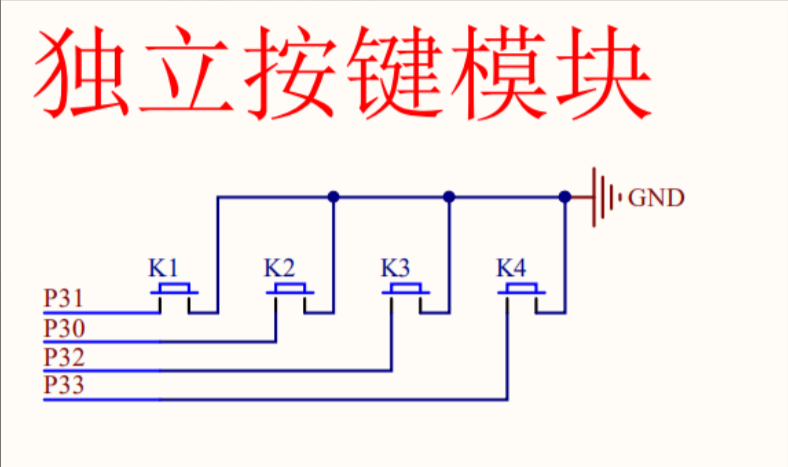# #冲刺创作新星#51单片机出现正在检测单片机的原因以及点灯测试的 原创

0收藏

51单片机是对兼容​​英特尔​​​8051​​指令系统​​​的​​单片机​​​的统称。51单片机广泛应用于家用电器、汽车、工业测控、通信设备中。因为51单片机的​​指令系统​​、内部结构相对简单，所以国内许多高校用其进行单片机入门教学。具体型号可以看自己的单片机上的丝印``````#include <REGX52.H>

void Delayxms(unsigned int		//@12.000MHz{
unsigned char i, j;
while(x--)
{
i = 12;
j = 169;
do
{
while (--j);
} while (--i);
}
}

void main(){
P2 = 0xFE;
while(1)
{
if(P3_1==0)
{
Delayxms(20);
while(P3_1==0);
Delayxms(20);
P2 = ~((~P2)>>1);
if(P2==0xFF)
{
P2=0x7F;
}
}
if(P3_0==0)
{
Delayxms(20);
while(P3_0==0);
Delayxms(20);
P2=~((~P2)<<1);
if(P2==0xFF)
{
P2=0xFE;
}
}
}
}``````

``````#include <REGX52.H>

void Delayxms(unsigned int		//@12.000MHz{
unsigned char i, j;
while(x--)
{
i = 2;
j = 239;
do
{
while (--j);
}while (--i);
}
}

void main(){
while(1)
{
if(P3_1==0)
{
Delayxms(20);
while(P3_1==0);
Delayxms(20);
P2--;
}
}
}``````